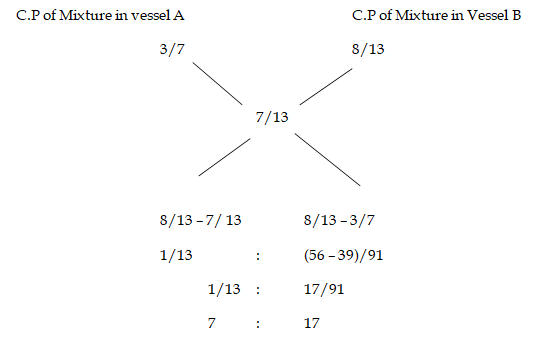# RRB ALP 2018 Practice Test Papers | Arithmetic Questions (Day-50)

Dear Aspirants, Here we have given the Important RRB ALP & Technicians Exam 2018 Practice Test Papers. Candidates those who are preparing for RRB ALP 2018 can practice these Arithmetic Questions to get more confidence to Crack RRB 2018 Examination.

[WpProQuiz 2662]

Click “Start Quiz” to attend these Questions and view Explanation

1. The perimeter of a square is 20 cm, find its area?
1. 20 cm2
2. 25 cm2
3. 40 cm2
4. 45 cm2
1. The average weight of 20 girls is 40kg and 30 boys are 60 kg in a hostel. Then what is the total Average weight of all boys and girls?
1. 45
2. 43
3. 40
4. 48
1. When a number is decreased by 12, it becomes 70% of itself. What is the number?
1. 50
2. 40
3. 30
4. 25
1. Two vessels A and B contain Milk and water in the ratio 3 : 4 and 8 : 5 respectively. Find the ratio in which these mixtures be mixed to obtain a new mixture in vessel C containing Milk and water in the ratio 7 : 6 ?
1. 9: 17
2. 17: 7
3. 7:17
4. 17

5.If the product of two numbers is 320 and the HCF is 8, then the ratio of HCF and LCM is

1. 1: 5
2. 5:1
3. 4: 1
4. 1: 4
1. What is the sum of all the natural numbers from 1 to 35?
1. 620
2. 650
3. 630
4. 640
1. Nikil has sold two mobiles at Rs.2500 each. On one, he gains 25% and on the other, he loses 25%. The gain or loss % on the whole transaction is:
1. 7.5% loss
2. 6.5 % gain
3. 6.25% loss
4. 7.75% gain
1. Two pipes, A can fill the tank in 15 minutes and b can fill a tan in 30 minutes respectively. If both are opened together and at the end of 6 minutes, A is closed, how much longer will the tank take to fill?
1. 3 minutes
2. 6 minutes
3. 9 minutes
4. 12 minutes
1. tan 45° + cot 45° = x, then find the value of x.
1. 0
2. 1
3. 2
4. √2
1. How many words can be formed by using all the letters of word ‘TASTE’?
1. 120
2. 24
3. 60
4. 720

Using the formulas

A=a2

P=4a

20 = 4a

a = 20÷4 = 5cm

Area = (a2) = 52 = 25cm2

Total weight of Girls = 20×40 = 800

Total weight of Boys = 30×60=1200

Average of Boys and girls = 2000/ 50 = 40

x – 12 = (70/100)x

100(x-12)= 70x

100x – 1200 = 70x

30x = 1200

X= 40Product of two numbers = LCM × HCF

⇒ 320 = LCM × 8

⇒ LCM = 40

Ratio of HCF to LCM = 8 : 40 = 1 : 5

Sum of n natural no. = n(1+n)/2

= 35×36/2

=35×18 = 630

Loss on total deal,
= (Common loss or gain percentage /10)2 = (25/10)2
= 6.25% loss

Pipe A = 1/15

Pipe B = 1/ 30

A+B = 1/15 + 1/30 = (1/10)*6 = 6/10 = 3/5 tank filled

Remaining tank will be filled by B

Remaining tank= ( 2/5 )*30 =2*6 =12 minutes

tan45 °= 1

cot 45° = 1

1+1 =2

5 letter word can be arranged in 5 different ways = 5! / 2

= 120/2 =60

RRB ALP 2018 – “All in One” Study Materials and Practice Sets